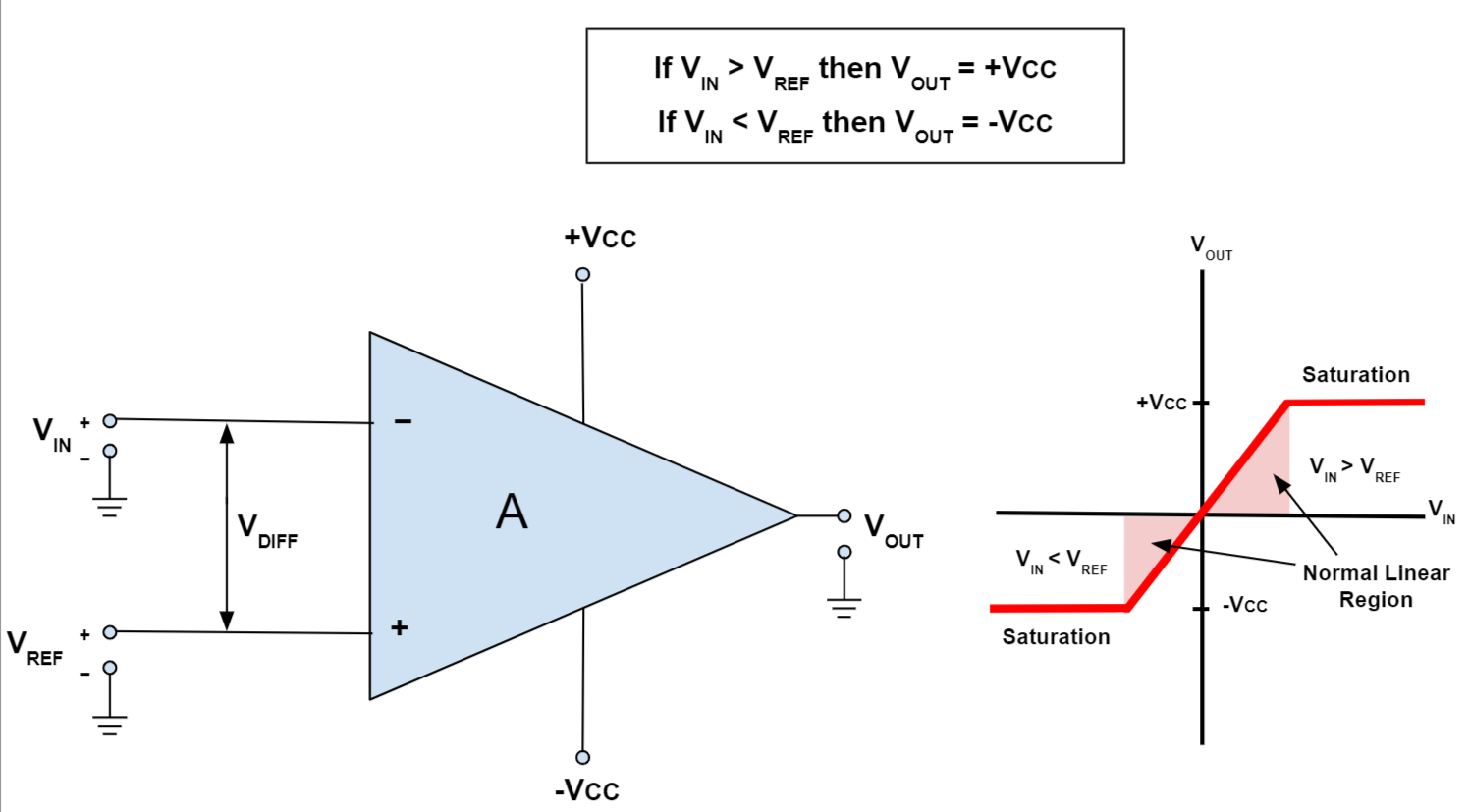# 运算放大器## 运算放大器的分类

• 吸收电压并在输出端产生电压的电压放大器。
• 接收电流输入并产生电流输出的电流放大器。
• 将电压输入转换为电流输出的跨导放大器。
• 将电流输入转换为电压输出的跨阻放大器。

## 运算放大器：关键特性和参数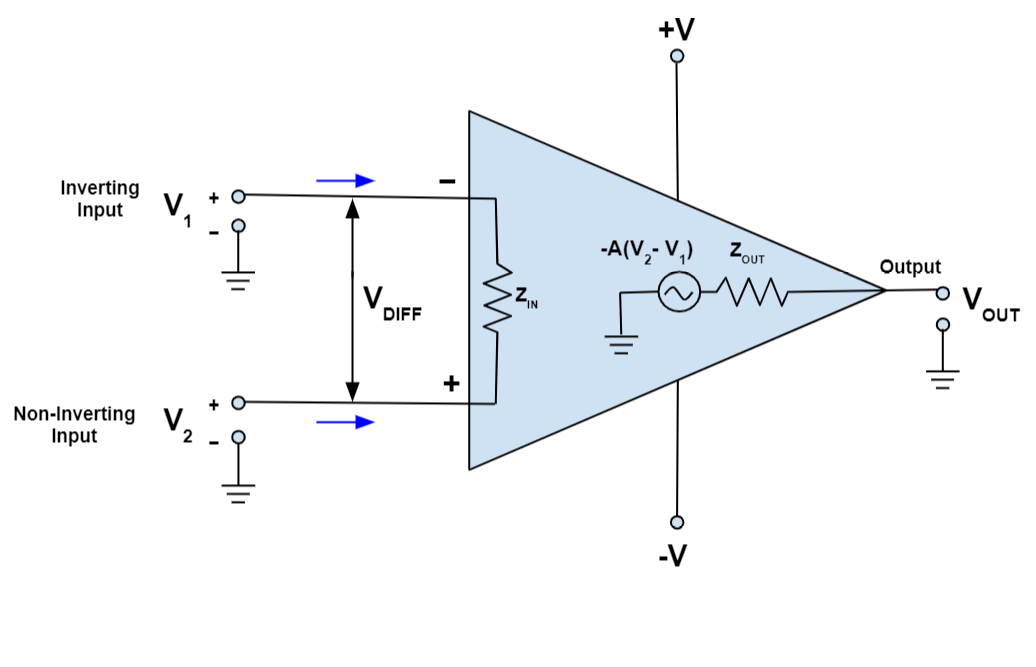### 频率响应和带宽（BW）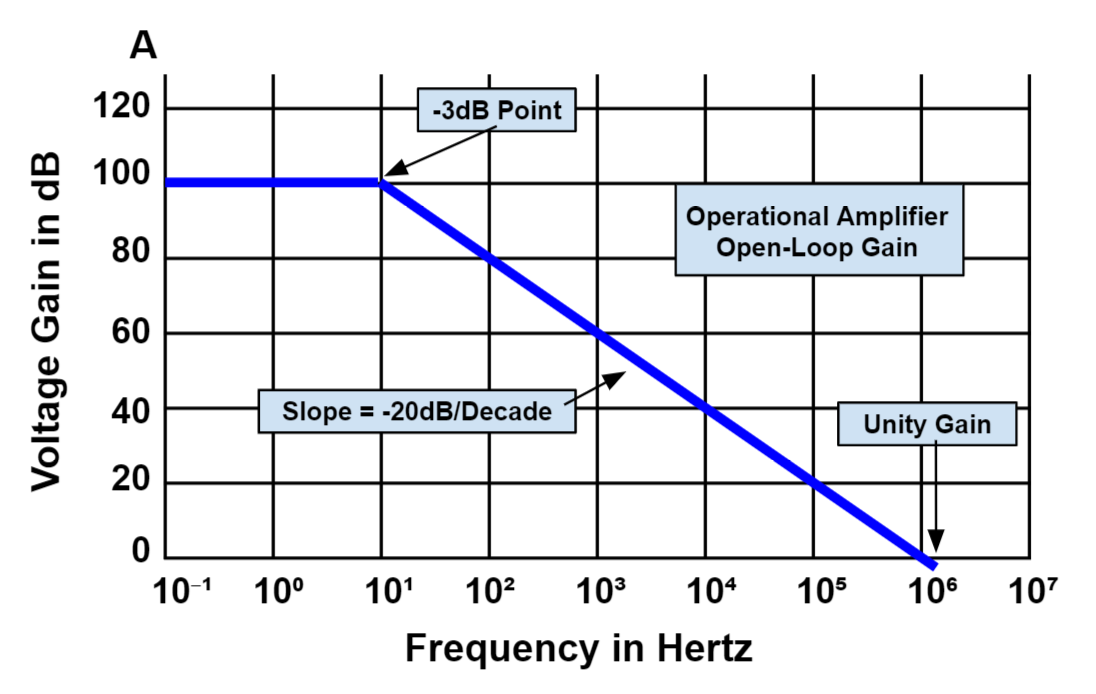### 增益带宽积（GBP）

$$GBP = 增益x带宽 = AxBW$$

GBP是在运算放大器的增益达到单位增益的频率点测得。用户可以用它来计算不同频率下设备的开环增益。GBP通常用于衡量一个运放是否有用并衡量其性能，因为具有较高GBP的运算放大器可在较高频率下提供更佳性能。

## 负反馈和闭环增益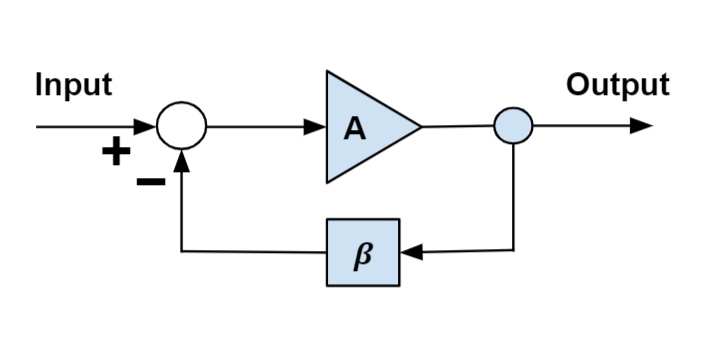$$\frac {V_{OUT}}{V_{IN}} = \frac 1 f$$

## 运算放大器配置拓扑

### 电压跟随器

$$V_{OUT} = V_{IN}$$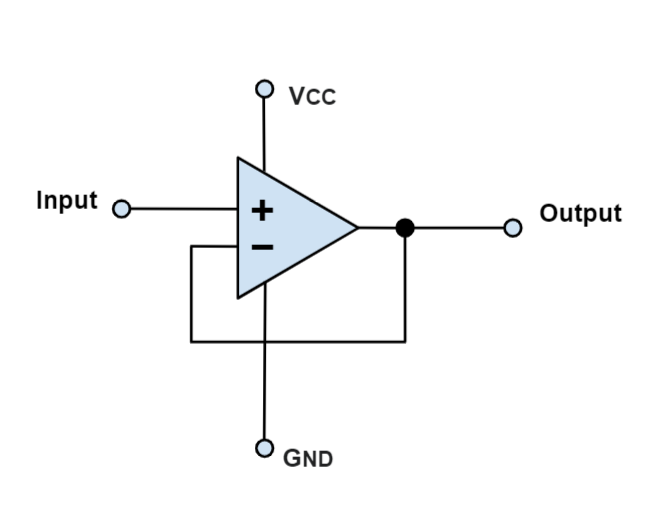### 反相运算放大器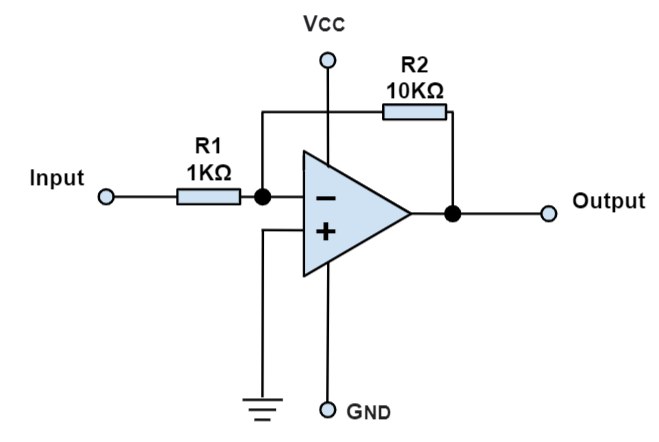$$V_{OUT} = - \left({R_2} \over {R_1}\right) x V_{IN}$$

### 同相运算放大器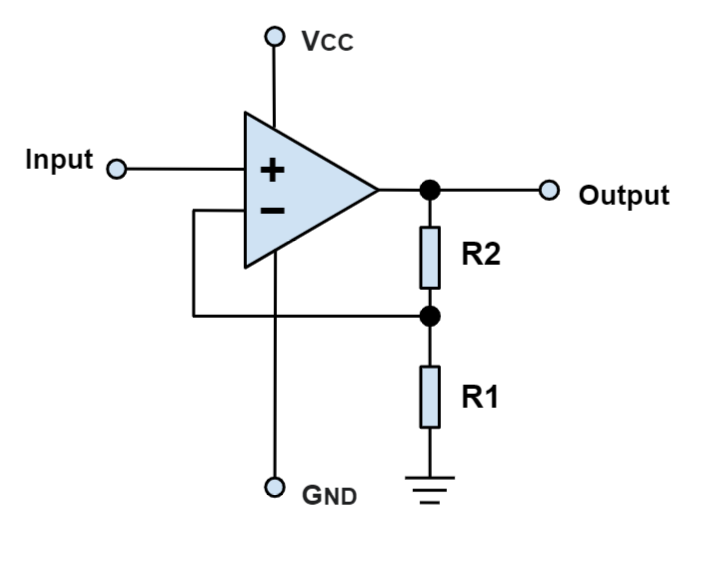$$V_{OUT} = \left(1 + \frac {R_1}\right) x V_{IN}$$

### 电压比较器